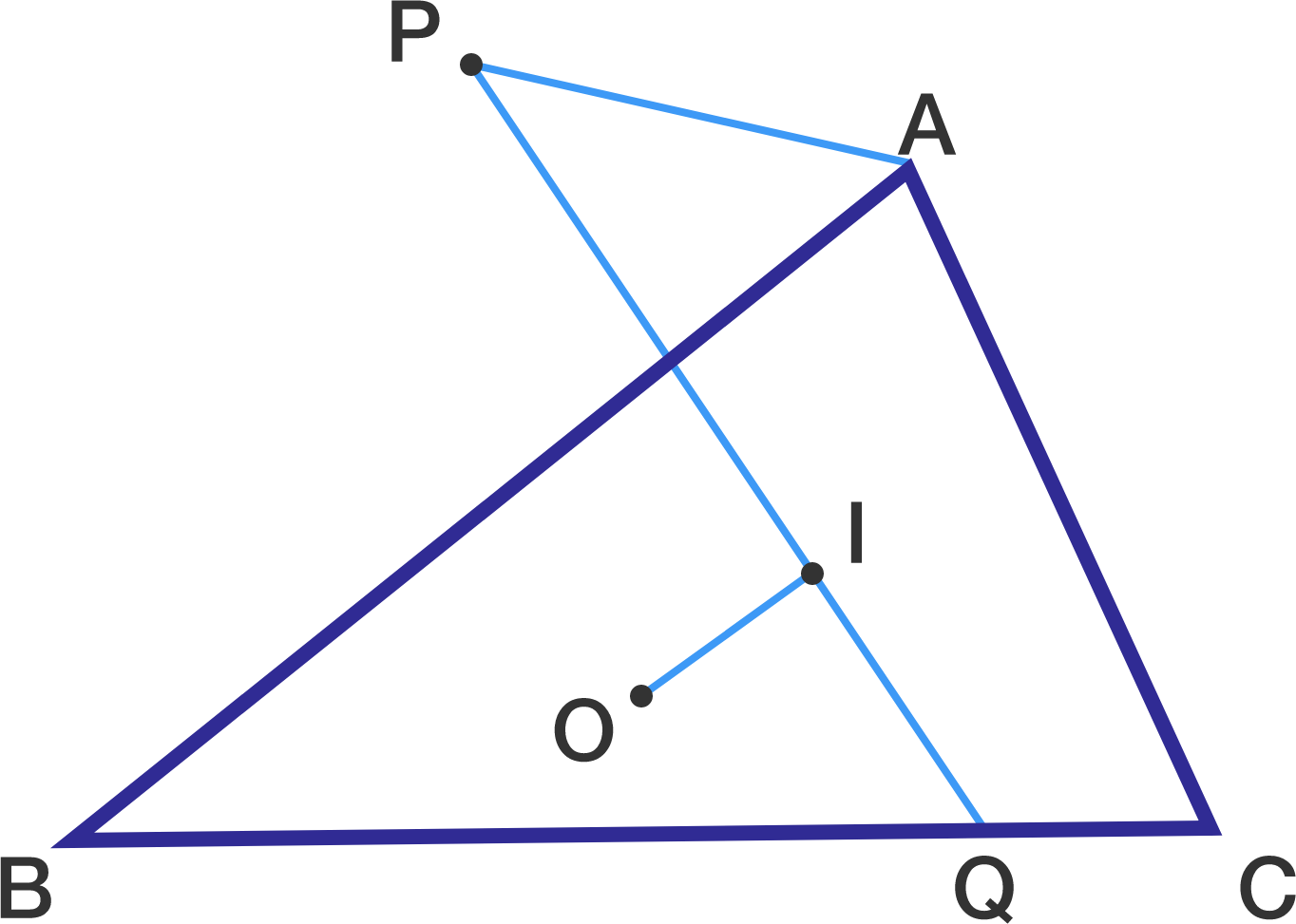# Two Triangles Centers At Play

Geometry Level 1

In triangle $ABC$, $O$ is the circumcenter and $I$ is the incenter. $P$ is a point on the exterior angle bisector of $\Delta BAC$ such that $PI \perp OI$. Extend $PI$ to intersect $BC$ at $Q$.

Find $\dfrac{PI}{QI}$.Note: Figure not drawn to scale.

×